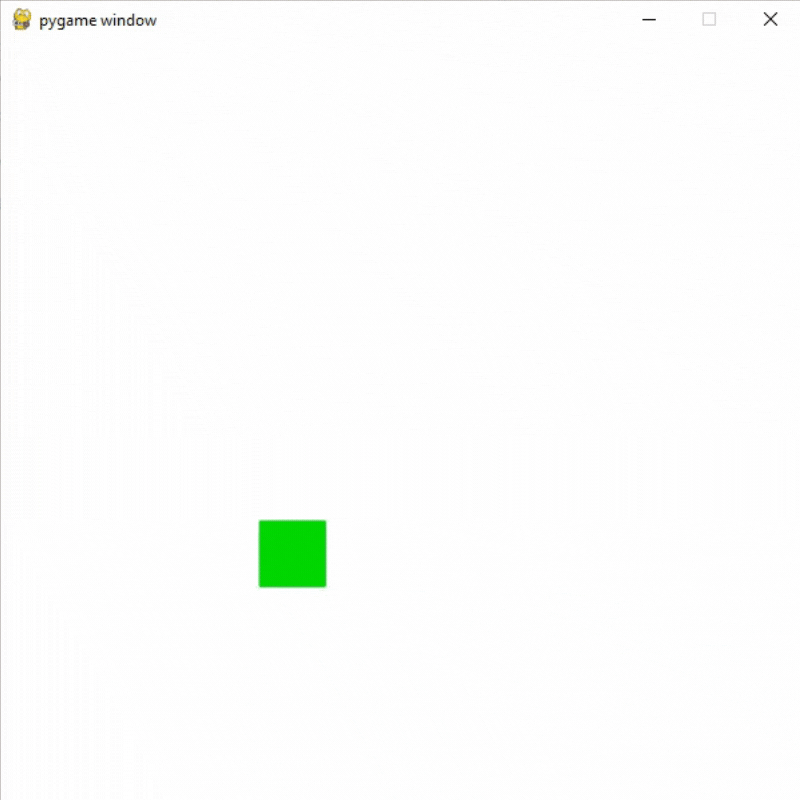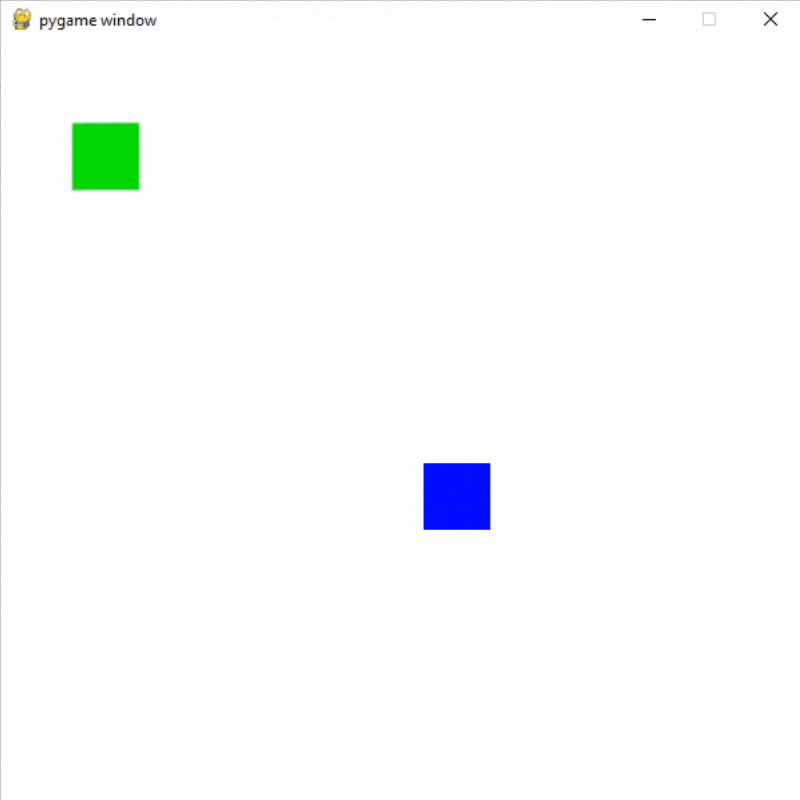Open in App
Not now

# Pygame – Random Movement of Object

• Difficulty Level : Medium
• Last Updated : 24 Sep, 2021

Prerequisite: How to draw rectangle in Pygame?

In this article, we will learn how we can add random movements in objects using PyGame in Python.

## Random movement in a single object

To add random movement in our object we are going to use randint method of the random module.

Syntax: random.randint(a, b)

Parameters:

a and b : Two integers

Returns: Return a random integer that lies between a and b.

First, a frame with necessary adjustments is created, then an object is placed on the window to depict movement. Now by using randint() and setting other relevant parameters we can change the position of the object randomly.

Example: Random movement in one object

## Python3

 `# Importing the pygame module``import` `pygame``from` `pygame.``locals` `import` `*` `# Import randint method random module``from` `random ``import` `randint` `# Initiate pygame and give permission``# to use pygame's functionality``pygame.init()` `# Create a display surface object``# of specific dimension``window ``=` `pygame.display.set_mode((``600``, ``600``))` `# Creating a new clock object to``# track the amount of time``clock ``=` `pygame.time.Clock()` `# Creating a variable for direction``direction ``=` `1` `# Creating a new rect for player``player_rect ``=` `Rect(``100``, ``100``, ``50``, ``50``)` `# Starting speed``speed_x ``=` `5``speed_y ``=` `4` `# Creating a boolean variable that``# we will use to run the while loop``run ``=` `True` `# Creating an infinite loop``# to run our game``while` `run:` `    ``# Setting the framerate to 60fps``    ``clock.tick(``60``)` `    ``# Changing the direction and x,y coordinate``    ``# of the object if the coordinate of left``    ``# side is less than equal to 20 or right side coordinate``    ``# is greater than equal to 580``    ``if` `player_rect.left <``=` `20` `or` `player_rect.right >``=` `580``:``        ``direction ``*``=` `-``1``        ``speed_x ``=` `randint(``0``, ``8``) ``*` `direction``        ``speed_y ``=` `randint(``0``, ``8``) ``*` `direction` `        ``# Changing the value if speed_x``        ``# and speed_y both are zero``        ``if` `speed_x ``=``=` `0` `and` `speed_y ``=``=` `0``:``            ``speed_x ``=` `randint(``2``, ``8``) ``*` `direction``            ``speed_y ``=` `randint(``2``, ``8``) ``*` `direction` `    ``# Changing the direction and x,y coordinate``    ``# of the object if the coordinate of top``    ``# side is less than equal to 20 or bottom side coordinate``    ``# is greater than equal to 580``    ``if` `player_rect.top <``=` `20` `or` `player_rect.bottom >``=` `580``:``        ``direction ``*``=` `-``1``        ``speed_x ``=` `randint(``0``, ``8``) ``*` `direction``        ``speed_y ``=` `randint(``0``, ``8``) ``*` `direction` `        ``# Changing the value if speed_x``        ``# and speed_y both are zero``        ``if` `speed_x ``=``=` `0` `and` `speed_y ``=``=` `0``:``            ``speed_x ``=` `randint(``2``, ``8``) ``*` `direction``            ``speed_y ``=` `randint(``2``, ``8``) ``*` `direction` `    ``# Adding speed_x and speed_y``    ``# in left and top coordinates of object``    ``player_rect.left ``+``=` `speed_x``    ``player_rect.top ``+``=` `speed_y` `    ``# Drawing player rect``    ``pygame.draw.rect(window, (``0``,   ``255``,   ``0``), player_rect)` `    ``# Updating the display surface``    ``pygame.display.update()` `    ``# Filling the window with white color``    ``window.fill((``255``, ``255``, ``255``))`

Output:## Random movement in more than one object

To add random movement in more than one object we are going to create separate speed and direction variables for the objects. Then we are going to check if the objects are colliding or not with the help of colliderect() method.

Syntax: pygame.Rect.colliderect(rect1 , rect2)

Parameters:

It will take two rects as its parameters.

Returns:

Returns true if any portion of either rectangle overlap.

Example: Random movement in two objects

## Python3

 `# Importing the pygame module``import` `pygame``from` `pygame.``locals` `import` `*` `# Import randint method random module``from` `random ``import` `randint` `# Initiate pygame and give permission``# to use pygame's functionality``pygame.init()` `# Create a display surface object``# of specific dimension``window ``=` `pygame.display.set_mode((``600``, ``600``))` `# Creating a new clock object to``# track the amount of time``clock ``=` `pygame.time.Clock()` `# Creating a variable for direction``# of object 1``direction_a ``=` `1` `# Creating a variable for direction``# of object 2``direction_b ``=` `1` `# Creating a new rect for first object``player_rect ``=` `Rect(``100``, ``100``, ``50``, ``50``)` `# Creating a new rect for second object``player_rect2 ``=` `Rect(``200``, ``200``, ``50``, ``50``)` `# Starting speed for object 1``speed_x1 ``=` `5``speed_y1 ``=` `4` `# Starting speed for object 2``speed_x2 ``=` `5``speed_y2 ``=` `4` `# Creating a boolean variable that``# we will use to run the while loop``run ``=` `True` `# Creating an infinite loop``# to run our game``while` `run:` `    ``# Setting the framerate to 60fps``    ``clock.tick(``60``)` `    ``# Changing the direction and x,y coordinate``    ``# of the object if the coordinate of left``    ``# side is less than equal to 20 or right side coordinate``    ``# is greater than equal to 580``    ``if` `player_rect.left <``=` `20` `or` `player_rect.right >``=` `580``:``        ``direction_a ``*``=` `-``1``        ``speed_x1 ``=` `randint(``0``, ``8``)``        ``speed_y1 ``=` `randint(``0``, ``8``)` `        ``# Changing the value if speed_x``        ``# and speed_y both are zero``        ``if` `speed_x1 ``=``=` `0` `and` `speed_y1 ``=``=` `0``:``            ``speed_x1 ``=` `randint(``2``, ``8``)``            ``speed_y1 ``=` `randint(``2``, ``8``)` `    ``# Changing the direction and x,y coordinate``    ``# of the object if the coordinate of top``    ``# side is less than equal to 20 or bottom side coordinate``    ``# is greater than equal to 580``    ``if` `player_rect.top <``=` `20` `or` `player_rect.bottom >``=` `580``:``        ``direction_a ``*``=` `-``1``        ``speed_x1 ``=` `randint(``0``, ``8``)``        ``speed_y1 ``=` `randint(``0``, ``8``)` `        ``# Changing the value if speed_x``        ``# and speed_y both are zero``        ``if` `speed_x1 ``=``=` `0` `and` `speed_y1 ``=``=` `0``:``            ``speed_x1 ``=` `randint(``2``, ``8``)``            ``speed_y1 ``=` `randint(``2``, ``8``)` `    ``# Changing the direction and x,y coordinate``    ``# of the object2 if the coordinate of left``    ``# side is less than equal to 20 or right side coordinate``    ``# is greater than equal to 580``    ``if` `player_rect2.left <``=` `20` `or` `player_rect2.right >``=` `580``:``        ``direction_b ``*``=` `-``1``        ``speed_x2 ``=` `randint(``0``, ``8``)``        ``speed_y2 ``=` `randint(``0``, ``8``)` `        ``# Changing the value if speed_x``        ``# and speed_y both are zero``        ``if` `speed_x2 ``=``=` `0` `and` `speed_y2 ``=``=` `0``:``            ``speed_x2 ``=` `randint(``2``, ``8``)``            ``speed_y2 ``=` `randint(``2``, ``8``)` `    ``# Changing the direction and x,y coordinate``    ``# of the object2 if the coordinate of top``    ``# side is less than equal to 20 or bottom side coordinate``    ``# is greater than equal to 580``    ``if` `player_rect2.top <``=` `20` `or` `player_rect2.bottom >``=` `580``:``        ``direction_b ``*``=` `-``1``        ``speed_x2 ``=` `randint(``0``, ``8``)``        ``speed_y2 ``=` `randint(``0``, ``8``)` `        ``# Changing the value if speed_x``        ``# and speed_y both are zero``        ``if` `speed_x2 ``=``=` `0` `and` `speed_y2 ``=``=` `0``:``            ``speed_x2 ``=` `randint(``2``, ``8``)``            ``speed_y2 ``=` `randint(``2``, ``8``)` `    ``# Adding speed_x1 and speed_y1``    ``# in left and top coordinates of object``    ``# ad multiplying with direction_a``    ``player_rect.left ``+``=` `speed_x1 ``*` `direction_a``    ``player_rect.top ``+``=` `speed_y1 ``*` `direction_a` `    ``# Adding speed_x2 and speed_y2``    ``# in left and top coordinates of object2``    ``# and multiplying with direction_b``    ``player_rect2.left ``+``=` `speed_x2 ``*` `direction_b``    ``player_rect2.top ``+``=` `speed_y2 ``*` `direction_b` `    ``# Checking if player is colliding``    ``# with platform or not using the``    ``# colliderect() method.``    ``# It will return a boolean value``    ``collide ``=` `pygame.Rect.colliderect(player_rect, player_rect2)` `    ``# If the objects are colliding``    ``# then changing their direction``    ``if` `collide:``        ``direction_a ``*``=` `-``1``        ``direction_b ``*``=` `-``1` `    ``# Drawing player rect``    ``pygame.draw.rect(window, (``0``,   ``255``,   ``0``), player_rect)``    ``# Drawing player rect2``    ``pygame.draw.rect(window, (``0``,   ``0``,   ``255``), player_rect2)` `    ``# Updating the display surface``    ``pygame.display.update()` `    ``# Filling the window with white color``    ``window.fill((``255``, ``255``, ``255``))`

Output:My Personal Notes arrow_drop_up